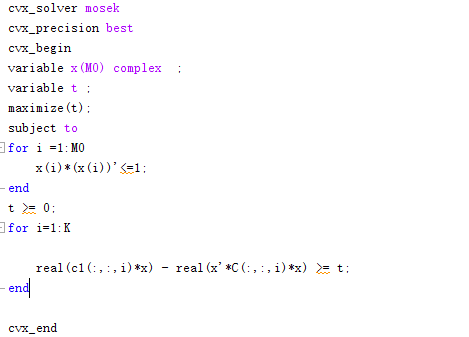# “Invalid quadratic form: not real.” How do I solve this error？

This is a max-min beamforming problem. The objective function is c1(:,:,i)*x - x’*C(:,:,i)*x (i = 1…K), which is a concave function. And C is semidefinite . But there is a error " Invalid quadratic form: not real." in the line of c1(:,:,i)*x - x’*C(:,:,i)*x (i = 1…K). I would be appreciate it if someone can help me!Maybe `C` is not exactly hernitian semidefinite. If it is within roundoff error of being hermitian semidefinite, you can do “hermitianize” it by `C = 0.5*(C + C')` or apply `real(....)` as you seem to have done.